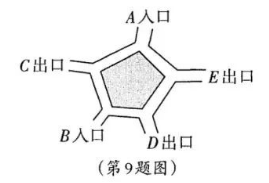$\text{A.}$ $\frac{2}{3}$ $\text{B.}$ $\frac{1}{2}$ $\text{C.}$ $\frac{1}{3}$ $\text{D.}$ $\frac{1}{6}$
【答案】 C

【解析】 系统有2个入口，3个出口，所以小颖和母亲从同一个出口出来的概率为 1/3，但是这个题目出的容易误解，在参观里，隐含着小颖和母亲一直没分开参观。